1594450146

# Graphs everywhere -  Finding the minimum swaps required to sort an array

Graphs are one of the most fundamental concepts in Computer Science. And they play the part perfectly in solving a tremendous number of problems. In this article, we’re going to bring into the light an algorithm question that at first glance one may not even think that graphs can be of any use to drive a solution for. But first, let’s have a look at our journey today. Please kindly note, we won’t go deep with the Graphs concepts in this article, rather, a shallow introduction over that and then to focus on its applications to tackle arrays algorithms questions.

1. A quick introduction to Graphs — Structure, Paths, and Cycles
2. The problem to solve — Finding the minimum required swaps to sort an array
3. Coding time
4. Always unit test your algorithms

## An Introduction to Graphs

1. The structure of a graph

A graph is a data structure that in its most compact form is made up of a single vertex (also called a node). Here’s a simple representation of it: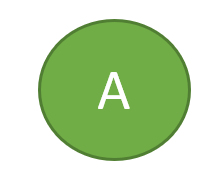Figure 1 — A single node graph

We can obviously extend this graph by adding more properties to it. For example, another connected node to this where the direction between the nodes is not important, just like the below: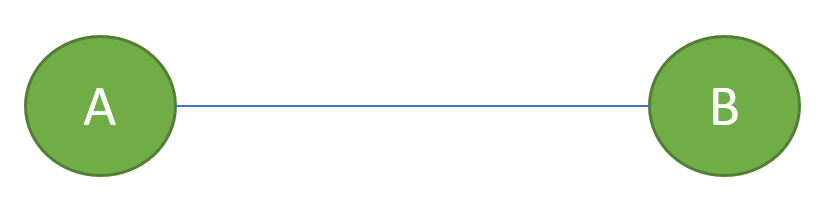Figure 2 — An undirected graph (direction does not matter)

Now, if we simply add a direction to the edge between the two nodes above, we would then turn it into a directed graph which also is important for the problem we are going to solve shortly. Here’s how it looks like: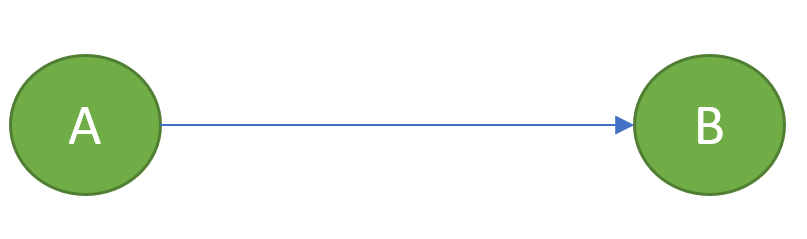Figure 2 — A directed graph (direction matters)

It really sounds easy so far, yet, we need to cover the Paths and Cycles of a graph so we get our backpack ready to solve our algorithm question.

_2. _Paths

To understand the path theoretically, we need to first have a touch on the Walks within a graph. So let’s see what that is:

**2.1. Walk **A Walk is basically a sequence of edges where each edge, except the first one, starts with a node (vertex) where the previous edge ended_. _For example, the following graph demonstrates a walk: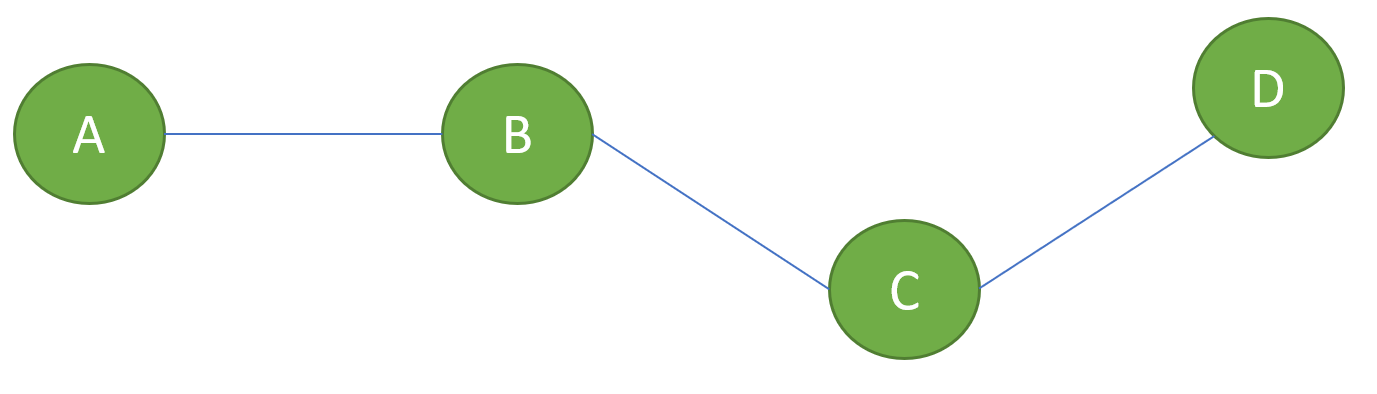Figure 3 — A walk on a graph

Here, if we traverse through A > B > C that would give us a walk — same if we travel A > B > C > D, or even, A > B > C > D > C.

The crucial note to remember here is that a Walk could have repeated edges and that would not have any interference with the definition of a Walk.

**2.2. Path — **A path is simply a walk where you would never go through an edge more than once. Hence, all the edges and vertices are distinct. Going back to the previous example, A > B > C would give us a path but not

A > B > C > D > C.

**2.3. Cycle — **A cycle can be detected when the _first _and the last node on a path are the same. That would give distinct edges but not distinct nodes which would lead to a Cycle. The following figure shows us a cycle of

A > B > C > D > A.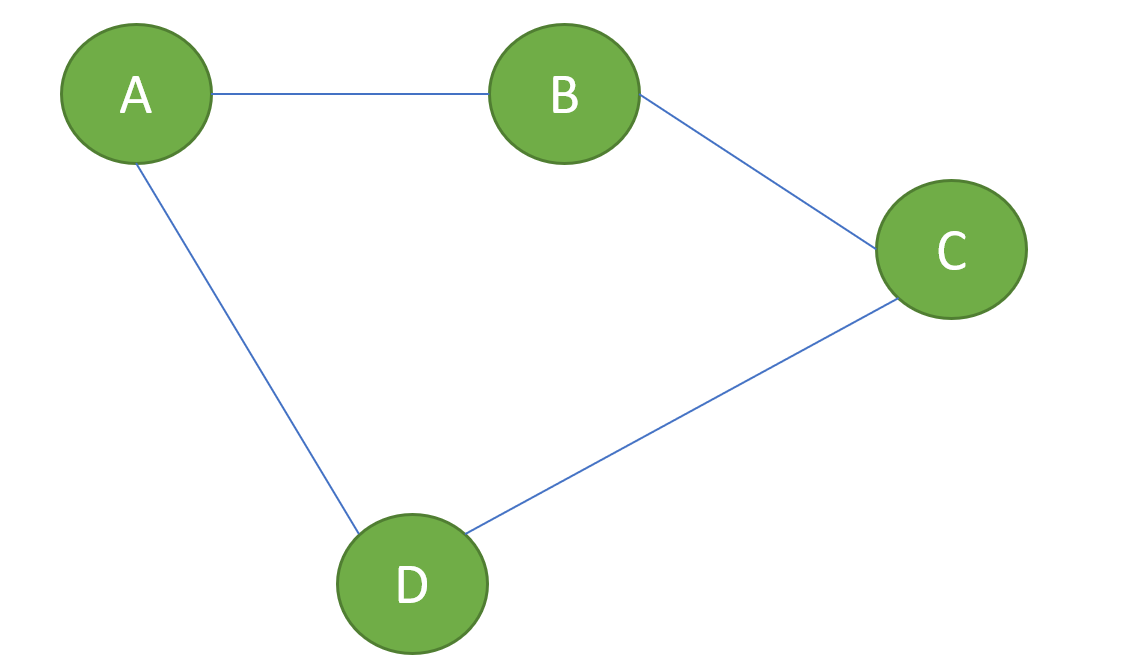Figure 4 — Representing a cycle for A > B > C > D > A

Up to this point, we have covered all we needed to know so we can tackle the algorithm question we have. So without any further details let’s read on to find out more about the question.

#arrays #graph #programming #algorithms

## Buddha Community1594450146

## Graphs everywhere -  Finding the minimum swaps required to sort an array

Graphs are one of the most fundamental concepts in Computer Science. And they play the part perfectly in solving a tremendous number of problems. In this article, we’re going to bring into the light an algorithm question that at first glance one may not even think that graphs can be of any use to drive a solution for. But first, let’s have a look at our journey today. Please kindly note, we won’t go deep with the Graphs concepts in this article, rather, a shallow introduction over that and then to focus on its applications to tackle arrays algorithms questions.

1. A quick introduction to Graphs — Structure, Paths, and Cycles
2. The problem to solve — Finding the minimum required swaps to sort an array
3. Coding time
4. Always unit test your algorithms

## An Introduction to Graphs

1. The structure of a graph

A graph is a data structure that in its most compact form is made up of a single vertex (also called a node). Here’s a simple representation of it:Figure 1 — A single node graph

We can obviously extend this graph by adding more properties to it. For example, another connected node to this where the direction between the nodes is not important, just like the below:Figure 2 — An undirected graph (direction does not matter)

Now, if we simply add a direction to the edge between the two nodes above, we would then turn it into a directed graph which also is important for the problem we are going to solve shortly. Here’s how it looks like:Figure 2 — A directed graph (direction matters)

It really sounds easy so far, yet, we need to cover the Paths and Cycles of a graph so we get our backpack ready to solve our algorithm question.

_2. _Paths

To understand the path theoretically, we need to first have a touch on the Walks within a graph. So let’s see what that is:

**2.1. Walk **A Walk is basically a sequence of edges where each edge, except the first one, starts with a node (vertex) where the previous edge ended_. _For example, the following graph demonstrates a walk:Figure 3 — A walk on a graph

Here, if we traverse through A > B > C that would give us a walk — same if we travel A > B > C > D, or even, A > B > C > D > C.

The crucial note to remember here is that a Walk could have repeated edges and that would not have any interference with the definition of a Walk.

**2.2. Path — **A path is simply a walk where you would never go through an edge more than once. Hence, all the edges and vertices are distinct. Going back to the previous example, A > B > C would give us a path but not

A > B > C > D > C.

**2.3. Cycle — **A cycle can be detected when the _first _and the last node on a path are the same. That would give distinct edges but not distinct nodes which would lead to a Cycle. The following figure shows us a cycle of

A > B > C > D > A.Figure 4 — Representing a cycle for A > B > C > D > A

Up to this point, we have covered all we needed to know so we can tackle the algorithm question we have. So without any further details let’s read on to find out more about the question.

#arrays #graph #programming #algorithms1603846800

## Merge two sorted arrays in O(1) extra space using Heap

Given two sorted arraysarr[]brr[] of size N, and M, the task is to merge the two given arrays such that they form a sorted sequence of integers combining elements of both the arrays.

Examples:

Input:_ arr[] = {10}, brr[] = {2, 3}_

Output: 2 3 10

Explanation:_ The merged sorted array obtained by taking all the elements from the both the arrays is {2, 3, 10}._

Therefore, the required output is 2 3 10.

Input:_ arr[]__= {1, 5, 9, 10, 15, 20}, brr[] = {2, 3, 8, 13}_

Output:_ 1 2 3 5 8 9 10 13 15 20_

Naive Approach: Refer to Merge two sorted arrays for the simplest approach to merge the two given arrays.

_Time Complexity: _O(N * M)

Auxiliary Space:_ O(1)_

Space Optimized Approach: Refer to Merge two sorted arrays with O(1) extra space to merge the two given arrays without using any extra memory.

Time Complexity:_ O(N * M)_

Auxiliary Space:_ O(1)_

Efficient Space Optimized Approach: Refer to Efficiently merging two sorted arrays with O(1) extra space to merge the two given array without using any extra memory.

Time Complexity:_ O((N + M) * log(N + M))_

Auxiliary Space:_ O(1)_

Heap** – based Approach:** The problem can be solved using Heap. The idea is to traverse arr[] array and compare the value of arr[i] with brr and check if arr[i] is greater than **brr **or not. If found to be true then swap(arr[i], brr[0) and perform the heapify operation on brr[]. Follow the steps below to solve the problem:

• Traverse the array arr[] and compare the value of arr[i] with brr and check if arr[i] is greater than brr or not. If found to be true then swap(arr[i], brr[0) and perform the heapify operation on brr[].
• Finally, sort the array brr[] and print both array.

Below is the implementation of the above approach:

• C++

`// C++ program to implement`

`// the above approach`

`#include <bits/stdc++.h>`

`**using**` `**namespace**` `std;`

`// Function to perform min heapify`

`// on array brr[]`

`**void**` `minHeapify(``**int**` `brr[],` `**int**` `i,` `**int**` `M)`

`{`

`// Stores index of left child`

`// of i.`

`**int**` `left = 2 * i + 1;`

`// Stores index of right child`

`// of i.`

`**int**` `right = 2 * i + 2;`

`// Stores index of the smallest element`

`// in (arr[i], arr[left], arr[right])`

`**int**` `smallest = i;`

`// Check if arr[left] less than`

`// arr[smallest]`

`**if**` `(left < M && brr[left] < brr[smallest]) {`

`// Update smallest`

`smallest = left;`

`}`

`// Check if arr[right] less than`

`// arr[smallest]`

`**if**` `(right < M && brr[right] < brr[smallest]) {`

`// Update smallest`

`smallest = right;`

`}`

`// if i is not the index`

`// of smallest element`

`**if**` `(smallest != i) {`

`// Swap arr[i] and arr[smallest]`

`swap(brr[i], brr[smallest]);`

`// Perform heapify on smallest`

`minHeapify(brr, smallest, M);`

`}`

`}`

`// Function to merge two sorted arrays`

`**void**` `merge(``**int**` `arr[],` `**int**` `brr[],`

`**int**` `N,` `**int**` `M)`

`{`

`// Traverse the array arr[]`

`**for**` `(``**int**` `i = 0; i < N; ++i) {`

`// Check if current element`

`// is less than brr`

`**if**` `(arr[i] > brr) {`

`// Swap arr[i] and brr`

`swap(arr[i], brr);`

`// Perform heapify on brr[]`

`minHeapify(brr, 0, M);`

`}`

`}`

`// Sort array brr[]`

`sort(brr, brr + M);`

`}`

`// Function to print array elements`

`**void**` `printArray(``**int**` `arr[],` `**int**` `N)`

`{`

`// Traverse array arr[]`

`**for**` `(``**int**` `i = 0; i < N; i++)`

`cout << arr[i] <<` `" "``;`

`}`

`// Driver Code`

`**int**` `main()`

`{`

`**int**` `arr[] = { 2, 23, 35, 235, 2335 };`

`**int**` `brr[] = { 3, 5 };`

`**int**` `N =` `**sizeof**``(arr) /` `**sizeof**``(arr);`

`**int**` `M =` `**sizeof**``(brr) /` `**sizeof**``(brr);`

`// Function call to merge`

`// two array`

`merge(arr, brr, N, M);`

`// Print first array`

`printArray(arr, N);`

`// Print Second array.`

`printArray(brr, M);`

`**return**` `0;`

`}`

Output:

``````2 3 5 23 35 235 2335
``````

Time Complexity:_ O((N + M) * log (M))_

Auxiliary Space:_ O(1)_

Efficient Approach: Refer to merge two sorted arrays to efficiently merge the two given arrays.

_Time Complexity: _O(N + M)

Auxiliary Space:_ O(N + M)_

Attention reader! Don’t stop learning now. Get hold of all the important DSA concepts with the DSA Self Paced Course at a student-friendly price and become industry ready.

#arrays #divide and conquer #heap #mathematical #sorting #amazon #amdocs #array-merge #brocade #goldman sachs #heap sort #juniper networks #priority-queue #snapdeal1603839600

## Merge two sorted arrays in O(1) extra space using QuickSort partition

Given two sorted arraysarr[]brr[] of size N, and M, the task is to merge the two given arrays such that they form a sorted sequence of integers combining elements of both the arrays.

Examples:

Input:_ arr[] = {10}, brr[] = {2, 3}_

Output: 2 3 10

Explanation:_ The merged sorted array obtained by taking all the elements from the both the arrays is {2, 3, 10}._

Therefore, the required output is 2 3 10.

Input:_ arr[]__= {1, 5, 9, 10, 15, 20}, brr[] = {2, 3, 8, 13}_

Output:_ 1 2 3 5 8 9 10 13 15 20_

Naive Approach: Refer to Merge two sorted arrays for the simplest approach to merge the two given arrays.

_Time Complexity: _O(N * M)

Auxiliary Space:_ O(1)_

Space Optimized Approach: Refer to Merge two sorted arrays with O(1) extra space to merge the two given arrays without using any extra memory.

Time Complexity:_ O(N * M)_

Auxiliary Space:_ O(1)_

Efficient Space Optimized Approach: Refer to Efficiently merging two sorted arrays with O(1) extra space to merge the two given array without using any extra memory.

Time Complexity:_ O((N + M) * log(N + M))_

Auxiliary Space:_ O(1)_

Partition** – based Approach: **The idea is to consider the (N + 1)th element of the final sorted array as a pivot element and perform the quick sort partition around the pivot element. Finally, store the first N smaller elements of the final sorted array into the array, arr[] and the last M greater elements of the final sorted array into the array, brr[] in any order and sort both these arrays separately. Follow the steps below to solve the problem:

1. Initialize a variable, say index to store the index of each element of the final sorted array.
2. Find the (N + 1)th elementof the final sorted array as a pivot element.
3. Perform the quick sort partition around the pivot element.
4. Finally, sort both the array arr[] and brr[] separately.

Below is the implementation of the above approach:

• C++

`// C++ program to implement`

`// the above approach`

`#include <bits/stdc++.h>`

`**using**` `**namespace**` `std;`

`// Function to perform the partition`

`// around the pivot element`

`**void**` `partition(``**int**` `arr[],` `**int**` `N,`

`**int**` `brr[],` `**int**` `M,`

`**int**` `Pivot)`

`{`

`// Stores index of each element`

`// of the array, arr[]`

`**int**` `l = N - 1;`

`// Stores index of each element`

`// of the array, brr[]`

`**int**` `r = 0;`

`// Traverse both the array`

`**while**` `(l >= 0 && r < M) {`

`// If pivot is`

`// smaller than arr[l]`

`**if**` `(arr[l] < Pivot)`

`l--;`

`// If Pivot is`

`// greater than brr[r]`

`**else**` `**if**` `(brr[r] > Pivot)`

`r++;`

`// If either arr[l] > Pivot`

`// or brr[r] < Pivot`

`**else**` `{`

`swap(arr[l], brr[r]);`

`l--;`

`r++;`

`}`

`}`

`}`

`// Function to merge`

`// the two sorted array`

`**void**` `Merge(``**int**` `arr[],` `**int**` `N,`

`**int**` `brr[],` `**int**` `M)`

`{`

`// Stores index of each element`

`// of the array arr[]`

`**int**` `l = 0;`

`// Stores index of each element`

`// of the array brr[]`

`**int**` `r = 0;`

`// Stores index of each element`

`// the final sorted array`

`**int**` `index = -1;`

`// Stores the pivot element`

`**int**` `Pivot = 0;`

`// Traverse both the array`

`**while**` `(index < N && l < N && r < M) {`

`**if**` `(arr[l] < brr[r]) {`

`Pivot = arr[l++];`

`}`

`**else**` `{`

`Pivot = brr[r++];`

`}`

`index++;`

`}`

`// If pivot element is not found`

`// or index < N`

`**while**` `(index < N && l < N) {`

`Pivot = arr[l++];`

`index++;`

`}`

`// If pivot element is not found`

`// or index < N`

`**while**` `(index < N && r < M) {`

`Pivot = brr[r++];`

`index++;`

`}`

`// Place the first N elements of`

`// the sorted array into arr[]`

`// and the last M elements of`

`// the sorted array into brr[]`

`partition(arr, N, brr,`

`M, Pivot);`

`// Sort both the arrays`

`sort(arr, arr + N);`

`sort(brr, brr + M);`

`// Print the first N elements`

`// in sorted order`

`**for**` `(``**int**` `i = 0; i < N; i++)`

`cout << arr[i] <<` `" "``;`

`// Print the last M elements`

`// in sorted order`

`**for**` `(``**int**` `i = 0; i < M; i++)`

`cout << brr[i] <<` `" "``;`

`}`

`// Driver Code`

`**int**` `main()`

`{`

`**int**` `arr[] = { 1, 5, 9 };`

`**int**` `brr[] = { 2, 4, 7, 10 };`

`**int**` `N =` `**sizeof**``(arr) /` `**sizeof**``(arr);`

`**int**` `M =` `**sizeof**``(brr) /` `**sizeof**``(brr);`

`Merge(arr, N, brr, M);`

`**return**` `0;`

`}`

Output:

``````1 2 4 5 7 9 10
``````

Time Complexity:_ O((N + M)log(N + M))_

_Auxiliary Space: _O(1)

Efficient Approach: Refer to merge two sorted arrays to efficiently merge the two given arrays.

_Time Complexity: _O(N + M)

Auxiliary Space:_ O(N + M)_

Attention reader! Don’t stop learning now. Get hold of all the important DSA concepts with the DSA Self Paced Course at a student-friendly price and become industry ready.

#arrays #divide and conquer #mathematical #sorting #amazon #amdocs #array-merge #brocade #goldman sachs #juniper networks #linkedin #partition #quick sort #snapdeal

1599645721

## Bubble Sort Algorithm Demystified

As Bubble Sort represents the basic foundations of sorting, we’ve tried to demystify this sorting algorithm.

#bubble-sort #sorting #sorting-algorithm #array #practice1597813200

## Minimum cost to sort an Array such that swapping X and Y costs XY

Given an array of **N **distinct positive integers. The task is to find the minimum cost to sort the given array. The cost of swapping two elements X and Y is X*Y.

Examples:

Input:_ arr[] = {8, 4, 5, 3, 2, 7}_

Output:_ 57_

Explanation:

Swap element at index 4 with index 5 – cost(arrarr) = (27) = 14,

Array becomes {8, 4, 5, 3, 7, 2}

then, swap element at index 0 with 5 – cost(arrarr) = (82) = 16,

Array becomes {2, 4, 5, 3, 7, 8}

then, swap element at index 2 with 3 – cost(arrarr) = (53) = 15,

Array becomes {2, 4, 3, 5, 7, 8}

then, swap element at index 1 with 2 – cost(arrarr) = (43) = 12,

Array becomes {2, 3, 4, 5, 7, 8}

Array is now sorted and total cost = 14+16+15+12 = 57.

Input:_ arr[] = {1, 8, 9, 7, 6}_

Output:_ 36_

### Recommended: Please try your approach on {IDE} first, before moving on to the solution.

Approach: The idea is that for sorting a cycle we have two choices either to use only the local minimum of the cycle or to use both local and overall minimum of the array. Choose the one swap element that gives a lower cost. Below are the steps:

1. Calculate the local minimum (say local_minimum) which is the minimum element in the present cycle and the overall minimum (say overall_minimum) which is the minimum element in the whole array.
2. Calculate and store the cost to sort the cycle (say cost1) by using only local minimum value.
3. Also, calculate and store the cost to sort the cycle (say cost2) by using both local minimum value and the overall minimum value.
4. Now the minimum cost to sort this cycle will be minimum of the costs cost1 and cost2. Add this cost to the total cost.

Below is the illustration for the array arr[] = {1, 8, 9, 7, 6}: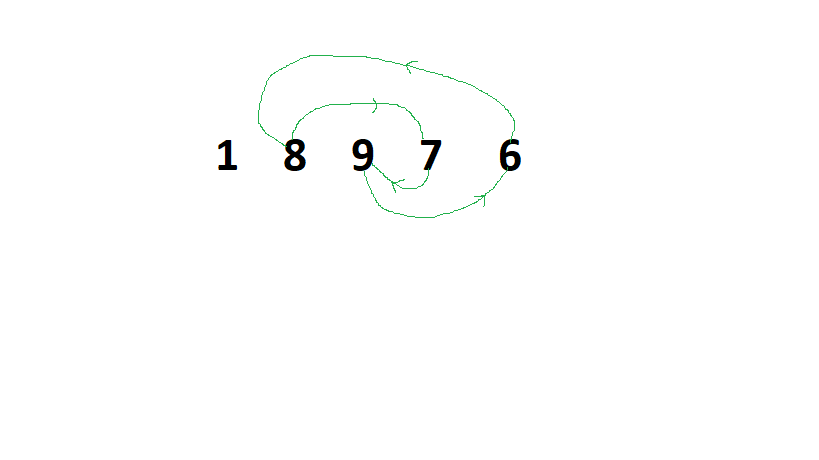• In the above figure, cycle {8, 9, 7, 6} can be sorted using the local minimum element 6 or with overall minimum element 1. By using only local minimum element i.e., swap 6 and 9, swap 6 and 7, swap 6 and 8. Therefore, the total cost is 69 + 67 + 6*8 = 144.
• By using both overall minimum and local minimum element i.e., swap 1 and 6, swap 1 and 9, swap 1 and 7, swap 1 and 8, swap 1 and 6. Therefore, the total cost is 16 +19 +17 +18 +1*6 = 36.
• The minimum of the above cost is 36.

Below is the implementation of the above approach:

• C++

`// C++ program for the above approach`

`#include <bits/stdc++.h>`

`**using**` `**namespace**` `std;`

`// Function returns the minimum cost`

`// to sort the given array`

`**int**` `minCost(``**int**` `arr[],` `**int**` `n)`

`{`

`// Create array of pairs in which`

`// 1st element is the array element`

`// and 2nd element is index of first`

`pair<``**int**``,` `**int**``> sorted[n];`

`// Initialize the total cost`

`**int**` `total_cost = 0;`

`**for**` `(``**int**` `i = 0; i < n; i++) {`

`sorted[i].first = arr[i];`

`sorted[i].second = i;`

`}`

`// Sort the array with respect to`

`// array value`

`sort(sorted, sorted + n);`

`// Initialize the overall minimum`

`// which is the 1st element`

`**int**` `overall_minimum = sorted.first;`

`// To keep track of visited elements`

`// create a visited array & initialize`

`// all elements as not visited`

`**bool**` `vis[n] = {` `**false**` `};`

`// Iterate over every element`

`// of the array`

`**for**` `(``**int**` `i = 0; i < n; i++) {`

`// If the element is visited or`

`// in the sorted position, and`

`// check for next element`

`**if**` `(vis[i] && sorted[i].second == i)`

`**continue**``;`

`// Create a vector which stores`

`// all elements of a cycle`

`vector<``**int**``> v;`

`**int**` `j = i;`

`// It covers all the elements`

`// of a cycle`

`**while**` `(!vis[j]) {`

`vis[j] =` `**true**``;`

`v.push_back(sorted[j].first);`

`j = sorted[j].second;`

`}`

`// If cycle is found then the`

`// swapping is required`

`**if**` `(v.size() > 0) {`

`// Initialize local minimum with`

`// 1st element of the vector as`

`// it contains the smallest`

`// element in the beginning`

`**int**` `local_minimum = v, result1 = 0,`

`result2 = 0;`

`// Stores the cost with using only`

`// local minimum value.`

`**for**` `(``**int**` `k = 1; k < v.size(); k++)`

`result1 += (local_minimum * v[k]);`

`// Stores the cost of using both`

`// local minimum and overall minimum`

`**for**` `(``**int**` `k = 0; k < v.size(); k++)`

`result2 += (overall_minimum * v[k]);`

`// Update the result2`

`result2 += (overall_minimum`

`* local_minimum);`

`// Store the minimum of the`

`// two result to total cost`

`total_cost += min(result1, result2);`

`}`

`}`

`// Return the minimum cost`

`**return**` `total_cost;`

`}`

`// Driver Code`

`**int**` `main()`

`{`

`// Given array arr[]`

`**int**` `arr[] = { 1, 8, 9, 7, 6 };`

`**int**` `n = (``**sizeof**``(arr) /` `**sizeof**``(``**int**``));`

`// Function Call`

`cout << minCost(arr, n);`

`**return**` `0;`

`}`

Output:

``````36
``````

Time Complexity: O(N)

Auxiliary Space: O(N)

Attention reader! Don’t stop learning now. Get hold of all the important DSA concepts with the DSA Self Paced Course at a student-friendly price and become industry ready.

#arrays #sorting #coding #css